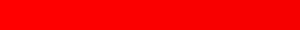# 基于图像的 LSB 隐写术科普

by

## 0x00 先放图LSB 算法鲁棒性比较低，窝建议泥通过这里下载原图 >ω<
（注：博客里使用的图片是直接引用的原图，所以你也可以右键保存）

## 0x02 LSB算法

### 通道

（摘自维基百科）

RGB，这里指灰度图像里的以 RGB 模式存储数字图像的模型。其中，R 是 Red 的首字母，G 是 Green 的首字母，B 是 Blue 的首字母，它们分别代表一个通道，在每个通道上，保存一个代表该通道亮度的数字，取值范围从 0 到 255 。

``````import javax.imageio.ImageIO;
import java.awt.*;
import java.awt.image.BufferedImage;
import java.io.*;

public class Main {
public static void main(String... args) throws IOException {
System.out.println(new Color(image.getRGB(0, 0)).toString());
}
}
``````

``````java.awt.Color[r=73,g=158,b=225]
``````

Java 返回了一个 `int`，为了方便观察，我们把它转为一个`java.awt.Color`对象。

### 肉眼难分的『色号』

``````import javax.imageio.ImageIO;
import java.awt.*;
import java.awt.image.BufferedImage;
import java.io.*;

public class Main {
private static void putPixel(BufferedImage image, int widthStart, int height, int rgb) {
for (int h = 0; h < height; ++h)
for (int i = 0; i < 30; i++) image.setRGB(widthStart + i, h, rgb);
}

public static void main(String... args) throws IOException {
int width = 30, height = 30;
BufferedImage img = new BufferedImage(width * 10, height, BufferedImage.TYPE_INT_RGB);
int r = 255, g = 0, b = 0;
for (int i = 0; i < 10; i++) {
putPixel(img, i * 30, height, new Color(r - i, g, b).getRGB());
}
ImageIO.write(img, "png", new File("tmp.png"));
}
}
``````（扯个皮，口红试色的时候，专柜的灯光比平时的灯光亮好多倍，所以会导致一些深的颜色看起来很浅，选口红的时候要注意哦，窝交了好些智商税才想明白这个道理 QAQ ）

## 0x03 开篇那张图

``````import javax.imageio.ImageIO;
import java.awt.*;
import java.awt.image.BufferedImage;
import java.io.File;
import java.io.IOException;

public class Main {
public static void main(String... args) throws IOException {
int width = secretImg.getWidth(), height = secretImg.getHeight();
for (int w = 0; w < width; w++) {
for (int h = 0; h < height; h++) {
Color color = new Color(secretImg.getRGB(w, h));
int lsb = color.getRed() % 2; // 个人建议把 % 2 写成 & 1
secretImg.setRGB(w, h, (lsb == 0 ? new Color(0, 0, 0) : new Color(255, 255, 255)).getRGB());
}
}
ImageIO.write(secretImg, "png", new File("secret.png"));
}
}
``````

``````public class Main {
public static void main(String... args) throws IOException {
for (int w = 0; w < secretImg.getWidth(); w++)
for (int h = 0; h < secretImg.getHeight(); h++) {
int lsb = new Color(secretImg.getRGB(w, h)).getRed() % 2; // 个人建议把 % 2 写成 & 1
secretImg.setRGB(w, h, (lsb == 0 ? new Color(0, 0, 0) : new Color(255, 255, 255)).getRGB());
}
ImageIO.write(secretImg, "png", new File("secret.png"));
}
}
``````

## 参考资料

• 用于隐写的原图的分享者为 Midia，原图地址
• 隐写术_维基百科
• 通道_维基百科
• 《数据隐藏技术揭秘》
• 《隐写分析原理与应用》

## 最后的最后

Tweet this
Top

Create an issue to apply for commentary